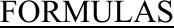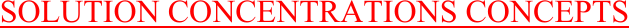Study Guides (380,000)
US (220,000)
Rutgers (3,000)
3:40 (100)
Final

01:160:161 Chapter Notes - Chapter 14: Colligative Properties, Mole Fraction, Boiling PointExam

Department
Chemistry
Course Code
01:160:161
Professor
Asbed Vassilia
Study Guide
Final

This preview shows pages 1-3. to view the full 75 pages of the document.1
PRACTICE PROBLEMS WITH DETAILED SOLUTIONS
CHAPTER 14 - PROPERTIES OF SOLUTIONS
SOLUTION CONCENTRATIONS CONCEPTS (Molarity, mole fraction, etc.)
SOLUTION CONCENTRATIONS CALCULATIONS (Molarity, mole fraction, etc.)
ENERGETICS OF SOLUTIONS AND SOLUBILITY CONCEPTS
ENERGETICS OF SOLUTIONS AND SOLUBILITY CALCULATIONS
VAPOR PRESSURE OF SOLUTIONS CONCEPTS (Henry’s Law, Raoult’s Law)
VAPOR PRESSURE OF SOLUTIONS CALCULATIONS
COLLIGATIVE PROPERTIES (BOILING POINT, FREEZING POINT, OSMOTIC PRESSURE
CHANGES) CONCEPTS
COLLIGATIVE PROPERTIES (BOILING POINT, FREEZING POINT, OSMOTIC PRESSURE
CHANGES) CALCULATIONS
MISCELLANEOUS (INCLUDING COLLOIDS)
E. Tavss, PhD

Only pages 1-3 are available for preview. Some parts have been intentionally blurred.2
FORMULAS
Mass percent = grams of solute/100 g solution
Molarity = moles of solute/L of solution
Molality = moles of solute/kilogram of solvent
PPM = grams of solute/1,000,000 grams solution
Volume percent = volume of solute/100 mL of solution
Proof = 2 x Vol. %; e.g., 2 x 40 mL/100 mL solution = 80 proof
Mole fraction : XA = nA/(nA + nB) XA + XB = 1
Particle fraction : PFXA = inA/(inA + inB) PFXA + PFXB = 1
Raoult’s law: Psoln = PFXsolventPo
solvent
PFXsolvent = insolvent/(insolvent + insolute)
PFXA + PFXB = 1
PTotal = PA + PB = PFXAPo
A + PFXBPo
B
van’t Hoff factor, “i”; i = moles of particles in solution/moles of solute dissolved
Boiling-point elevation: ΔT = Tf – Ti = Kbimsolute
Freezing-point depression: ΔT = Tf – Ti = -Kfimsolute
(+Kf gives absolute change in temp; -Kf gives actual Tf or Ti)
Osmotic pressure: πV = inRT
π = (n/V)iRT = iMsoluteRT
ΔHsoln = ΔHsolute-solutebondbreaking + ΔHsolvent-solventbondbreaking + ΔHsolute-solventbondforming
ΔHhydr = ΔHsolvent-solventbondbreaking + ΔHsolute-solventbondforming
ΔHsoln = ΔHsolute-solutebondbreaking + ΔHhydr
Henry’s Law: XA=kPA; XA = mole fraction of dissolved gas in solution

Only pages 1-3 are available for preview. Some parts have been intentionally blurred.3
SOLUTION CONCENTRATIONS CONCEPTS
CHEM 162-2010 EXAM I
Chapter 12 – Properties of Solutions
Solution concentrations concepts (molarity, mole fraction, etc.)
7. A solution can be carefully prepared to hold more solute than the maximum solubility. Such a solution
is called
A. supersaturated
B. subsaturated
C. saturated
D. isotonic
E. hypertonic
A. True. A supersaturated solution is one that contains more solute than is present in a saturated solution.
B. False. A subsaturated solution is one that contains less solute than is present in a saturated solution.
C. False. A saturated solution is one that contains the maximum amount of solute, while at equilibrium
with undissolved solute.
D. False. An isotonic solution is one that has the same osmotic pressure as body fluids.
E. False. A hypertonic solution is one that has an osmotic pressure greater than that of body fluids.
CHEM 162-2009 EXAM I
CHAPTER 12 – PROPERTIES OF SOLUTIONS
Solution concentrations concepts (Molarity, mole fraction, etc.)
21. Which of the following statements is true regarding molality and molarity?
A. Neither molality nor molarity depend on temperature.
B. Both molality and molarity depend on temperature.
C. Molarity depends on temperature but molality does not.
D. Molality depends on temperature but molarity does not.
E. Temperature dependence of molality and molarity depends on the material.
Molarity is moles of solute per liter of solution. If the solution is heated, the density decreases, resulting
in the same number of moles but an increase in volume of the solution. That is, if the temperature is
increased, the concentration of solute in solution (i.e., molarity) decreases. Therefore, molarity is
dependent on temperature. The purpose of the development of the molality concentration, was to have a
concentration which is temperature independent. That is, since molality is moles of solute per kg solvent,
and neither moles nor kg is affected by temperature, then molality is independent of temperature change.
A. False. Molality doesn’t depend on temperature, but molarity does.